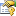﻿ BaseDiscriminantAnalysis Class# BaseDiscriminantAnalysis Class

Base class for Discriminant Analysis (LDA, QDA or KDA).Inheritance Hierarchy
SystemObject
Accord.MachineLearningTransformBaseDouble, Double
Accord.Statistics.AnalysisBaseDiscriminantAnalysis
Accord.Statistics.AnalysisKernelDiscriminantAnalysis
Accord.Statistics.AnalysisLinearDiscriminantAnalysis

Namespace:  Accord.Statistics.Analysis
Assembly:  Accord.Statistics (in Accord.Statistics.dll) Version: 3.8.0Syntax
```[SerializableAttribute]
public abstract class BaseDiscriminantAnalysis : TransformBase<double[], double[]>```

The BaseDiscriminantAnalysis type exposes the following members.Constructors
NameDescriptionBaseDiscriminantAnalysis
Initializes a new instance of the BaseDiscriminantAnalysis class
TopProperties
NameDescriptionClassCount
Gets the observation count for each class.Classes
Gets information about the distinct classes in the analyzed data.Classifications Obsolete.
Gets the original classifications (labels) of the source data given on the moment of creation of this analysis object.ClassMeans
Gets the Mean vector for each class.ClassScatter
Gets the Scatter matrix for each class.ClassStandardDeviations
Gets the Standard Deviation vector for each class.CumulativeProportions
The cumulative distribution of the discriminants factors proportions. Also known as the cumulative energy of the first dimensions of the discriminant space or as the amount of variance explained by those dimensions.DiscriminantMatrix Obsolete.
Gets the Eigenvectors obtained during the analysis, composing a basis for the discriminant factor space.DiscriminantProportions
Gets the level of importance each discriminant factor has in discriminant space. Also known as amount of variance explained.Discriminants
Gets the discriminant factors in a object-oriented fashion.DiscriminantVectors
Gets the Eigenvectors obtained during the analysis, composing a basis for the discriminant factor space.Eigenvalues
Gets the Eigenvalues found by the analysis associated with each vector of the ComponentMatrix matrix.Means
Gets the mean of the original data given at method construction.NumberOfClasses
Gets the number of classes in the analysis.NumberOfInputs
Gets the number of inputs accepted by the model.
(Inherited from TransformBaseTInput, TOutput.)NumberOfOutputs
Gets the number of outputs generated by the model.
(Inherited from TransformBaseTInput, TOutput.)NumberOfSamples
Gets the number of samples used to create the analysis.ProjectionMeans
Gets the feature space mean of the projected data.Result Obsolete.
Gets the resulting projection of the source data given on the creation of the analysis into discriminant space.ScatterBetweenClass
Gets the Between-Class Scatter Matrix for the data.ScatterMatrix
Gets the Total Scatter Matrix for the data.ScatterWithinClass
Gets the Within-Class Scatter Matrix for the data.Source Obsolete.
Returns the original supplied data to be analyzed.StandardDeviations
Gets the standard mean of the original data given at method construction.Threshold
Gets or sets the minimum variance proportion needed to keep a discriminant component. If set to zero, all components will be kept. Default is 0.001 (all components which contribute less than 0.001 to the variance in the data will be discarded).Token
Gets or sets a cancellation token that can be used to stop the learning algorithm while it is running.
TopMethods
NameDescriptionClassify(Double) Obsolete.
Classifies a new instance into one of the available classes.Classify(Double) Obsolete.
Classifies new instances into one of the available classes.Classify(Double, Double) Obsolete.
Classifies a new instance into one of the available classes.CreateDiscriminantsDiscriminantFunction
Gets the output of the discriminant function for a given class.Equals
Determines whether the specified object is equal to the current object.
(Inherited from Object.)Finalize
Allows an object to try to free resources and perform other cleanup operations before it is reclaimed by garbage collection.
(Inherited from Object.)GetHashCode
Serves as the default hash function.
(Inherited from Object.)GetNonzeroEigenvalues
Returns the number of discriminant space dimensions (discriminant factors) whose variance is greater than a given threshold.GetNumberOfDimensions
Returns the minimum number of discriminant space dimensions (discriminant factors) required to represent a given percentile of the data.GetType
Gets the Type of the current instance.
(Inherited from Object.)init Obsolete.
Obsolete.Init
Initializes common properties.MemberwiseClone
Creates a shallow copy of the current Object.
(Inherited from Object.)ToString
Returns a string that represents the current object.
(Inherited from Object.)Transform(Double) Obsolete.
Obsolete.Transform(Double)
Applies the transformation to an input, producing an associated output.
(Overrides TransformBaseTInput, TOutputTransform(TInput).)Transform(Double)
Applies the transformation to an input, producing an associated output.
(Overrides TransformBaseTInput, TOutputTransform(TInput).)Transform(TInput, TOutput)
Applies the transformation to an input, producing an associated output.
(Inherited from TransformBaseTInput, TOutput.)Transform(Double, Int32) Obsolete.
Obsolete.Transform(Double, Int32) Obsolete.
Obsolete.Transform(Double, Int32) Obsolete.
Obsolete.
TopExtension Methods
NameDescriptionHasMethod
Checks whether an object implements a method with the given name.
(Defined by ExtensionMethods.)IsEqual
Compares two objects for equality, performing an elementwise comparison if the elements are vectors or matrices.
(Defined by Matrix.)To(Type)Overloaded.
Converts an object into another type, irrespective of whether the conversion can be done at compile time or not. This can be used to convert generic types to numeric types during runtime.
(Defined by ExtensionMethods.)ToTOverloaded.
Converts an object into another type, irrespective of whether the conversion can be done at compile time or not. This can be used to convert generic types to numeric types during runtime.
(Defined by ExtensionMethods.)
TopSee Also